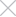We track sessions with cookies For what?Select a languageSeptember 14, 2022#tech

# Part 1. How do navigation apps plot the shortest path?

Has anyone ever wondered how modern navigation applications like 2GIS, Google Maps or Yandex.Maps plot routes from point A to point B? At the same time, such applications try to choose the shortest route for you.

In this article, I will tell you the basic algorithms on which all these applications are based when building any routes. And so, let's start.

For the most part, navigation systems use such a data structure as a “graph” to build routes. In short, a graph is a data structure that describes relationships between objects using nodes and edges. A node can be directly connected to several other nodes. These nodes are called neighbors. There are two types of graph. It is directional and non-directional.

In a directed graph, relations act only in one direction. In this example, node B is a neighbor of A, but node A is not a neighbor of node B. There are no arrows in an undirected graph, and each of the nodes is a neighbor with respect to each other. Basically, that's all there is to know about graphs.

And so, let's continue. Suppose we have two nodes: A and B, let it be our Home and Work, respectively. Let's say you are at Home and want to get to Work. You intend to travel by bus with a minimum number of transfers. Let's try to visualize the received information in the form of a graph. The following options are possible:

A problem of this type is a shortest path problem. It is often necessary to find a certain shortest path: the path to your friend's house, the path to victory in a chess game (in the least number of moves), etc. The algorithm for solving the problem of finding the shortest path is called a breadth-first search.

In our case, the shortest path is determined by the least number of transfers. Is it possible to get to node B without a transfer? Alas, no. We will only get to nodes C and D without transfers.

And with one transfer? Yes, the route with one transfer looks like this:

We will assume that the nodes that can be reached in 1 move are the connections of the first level, the nodes that can be reached in 2 moves are the connections of the second level, etc.

First-level links are preferable to second-level links, second-level links are preferable to third-level links, etc. It follows that the search for the nodes of the second level should not be performed until we have bypassed all the nodes of the first level and make sure that it is impossible to get to the endpoint B in 1 move.

This can also be explained by the fact that the first-level links are added to the search before the second-level links. Queues are great for implementing this approach. The queue belongs to the category of FIFO (First In, First Out) data structures.

Note that node B is both a second-level node and a third-level node. This is due to the fact that it can be reached in a different number of moves.

Let's move on to implementing a breadth-first search algorithm. First of all, we need to connect all nodes with all their neighbors. Hash tables are great for this. I will write in JS, because it is more common and intuitive:

`const graph = { `

` A: ['C', 'D'], `

` B: [], `

` C: ['B'], `

` D: ['E'],`

` E: ['B'], `

`} `

After that, we need to create a queue. Since there is no special object in JS designed to create queues, as it is, for example, implemented in Python or Java, I will use a regular array and its methods. Well, now we can implement the algorithm itself:

const node_is_endpoint = (node) => {

if (node === 'B') { <------ there can be any check here

return true

}

return false

const search = (graph, startNode) => {

const search_queue = [] <------ create a queue

search_queue.unshift(...graph[startNode]) <------ add the start node to the queue

const searched = [] <------- checked nodes do not need to be checked again.

while (search_queue.length) {

const node = search_queue.shift() <------- remove the first node from the queue

if (!searched.includes(node)) {

if (node_is_endpoint(node)) { <------- check if this is endpoint

console.log(`We are on place!`)

return true

} else {

search_queue.push(...graph[node]) <------- add all neighbors of this node

searched.push(node)

}

}

}

return false

That's all, we have implemented a breadth-first search algorithm.

P.S. But what if our graph looks like this?

Oh, and is this path exactly the shortest? And no breadth-first search will help us here. To help solve such a problem, there is a Dijkstra algorithm. But we will analyze it in the next part.